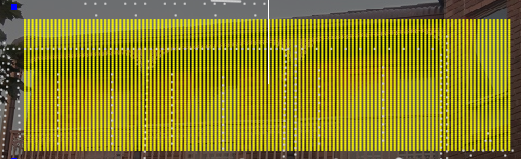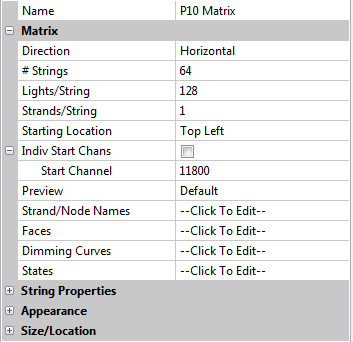Matrix Model

## Matrix ModelThe direction can be defined as horizontal (the most common) or vertical. The ‘# Strings’ refers to the number of vertical columns of the matrix and the Lights/string refers to the number of nodes in each horizontal row.
The starting location indicates where the matrix is connected to the controller (or where channel 1 of the matrix is).This is an example of a horizontal matrix used to define a P10 panel matrix.
Each panel is (32 W x 16 H) pixels and the panel matrix is 8 panels in landscape. The Start channel number is 11800.
For P10 panel matrices run off the BBB using the falcon player, , the matrix must always be defined as a horizontal matrix starting at the top left corner, irrespective of how the panels are physically oriented.